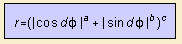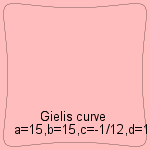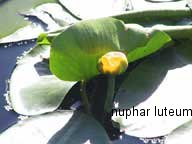# Gielis curve

## power

last updated: 2005-09-02The curve has been derived from the super ellipse by the Belgian botanist Johan Gielis, .
He converted the equation of this ellipse into polar coordinates and added a power to the radius (c).
He also added a parameter (d) to the angle to use more symmetries than the four from the Cartesian system. The number of symmetries is equal to 4 d.

You can imagine that with these four parameters an overwhelming lot of curves can be created.
Gielis states that "Many geometrical forms, both in nature and culture, can be interpreted as modified circles".
The formula is called a geometric superformula.

Some examples mentioned by Johan Gielis:

• a=10, b=10, c=-2/9, d=3/4: has the shape of the petiole (leaf stem) of the nuphar luteum
• a=15, b=15, c=-1/12, d=1: the shape of the cross section of the stem of the herb called scrophularia nodosa
• a=1, b=1, c=-1/4, d=5/4: idem, of the equisetum
• a=6, b=6, c=-1/10, d=7/4, idem, of the raspberryIn fact in nature one commonly sees a=b.

Plus the corresponding herbs:As long as the parameters a, b, c and d are rational numbers the curve is algebraic.

In the same way as these curves are derived from the ellipse/circle, super curves be derived from other polar curves, to get e.g. super roses and super spirals.